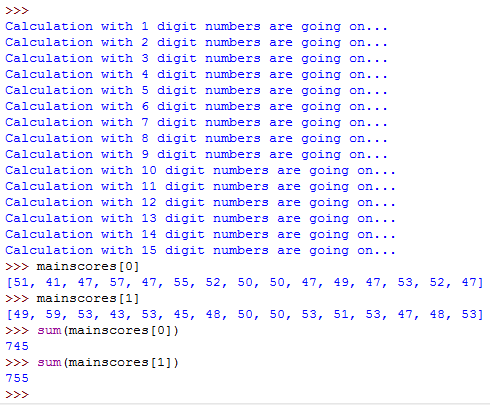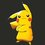# An experiment

Hello, I just thought of comparing the speeds of two methods to double a number in python but didn't got a precise result. Can anyone help me improving this testing?

Here's the program:

  1 2 3 4 5 6 7 8 9 10 11 12 13 14 15 16 17 18 19 20 21 22 23 24 25 import time,random mainscores=[[],[]] for digits in range(15): print('Calculations with %s digit numbers are going on...' %(digits+1)) scoreplus=0 scoremultiply=0 for m in range(100): timebyaplusa=0 timebyatimestwo=0 while timebyaplusa==timebyatimestwo: a=random.randint((10**digits),(10**(digits+1))-1) starttime=time.time() b1=a+a timebyaplusa+=time.time()-starttime starttime=time.time() b2=a*2 timebyatimestwo+=time.time()-starttime if b1!=b2: print('Error') if timebyaplusa>timebyatimestwo: scoremultiply+=1 else: scoreplus+=1 mainscores.append(scoreplus) mainscores.append(scoremultiply) 

...and here's the output:Will it even show any difference?Note by Pranjal Jain
6 years, 3 months ago

This discussion board is a place to discuss our Daily Challenges and the math and science related to those challenges. Explanations are more than just a solution — they should explain the steps and thinking strategies that you used to obtain the solution. Comments should further the discussion of math and science.

When posting on Brilliant:

• Use the emojis to react to an explanation, whether you're congratulating a job well done , or just really confused .
• Ask specific questions about the challenge or the steps in somebody's explanation. Well-posed questions can add a lot to the discussion, but posting "I don't understand!" doesn't help anyone.
• Try to contribute something new to the discussion, whether it is an extension, generalization or other idea related to the challenge.

MarkdownAppears as
*italics* or _italics_ italics
**bold** or __bold__ bold
- bulleted- list
• bulleted
• list
1. numbered2. list
1. numbered
2. list
Note: you must add a full line of space before and after lists for them to show up correctly
paragraph 1paragraph 2

paragraph 1

paragraph 2

[example link](https://brilliant.org)example link
> This is a quote
This is a quote
    # I indented these lines
# 4 spaces, and now they show
# up as a code block.

print "hello world"
# I indented these lines
# 4 spaces, and now they show
# up as a code block.

print "hello world"
MathAppears as
Remember to wrap math in $$ ... $$ or $ ... $ to ensure proper formatting.
2 \times 3 $2 \times 3$
2^{34} $2^{34}$
a_{i-1} $a_{i-1}$
\frac{2}{3} $\frac{2}{3}$
\sqrt{2} $\sqrt{2}$
\sum_{i=1}^3 $\sum_{i=1}^3$
\sin \theta $\sin \theta$
\boxed{123} $\boxed{123}$

Sort by:

Don't know anything about Python, but my guess is that only 1 trial per an $n$ digit number won't tell you much, since both methods have comparable times and the random factors obfuscate any differences in the short term.

If you want to see a difference, try more trials (1000?) and average them out instead of just one trial.

- 6 years, 3 months ago

Good idea! Let me try that out.

- 6 years, 3 months ago

Well, for 10k calculations, for $n$ digit numbers, $n\in \{1,2,3,\cdots,20\}$, the results were as follows:while with 1m calculations, the results were:Seems like '+' wins in case of bigger numbers.

- 6 years, 3 months ago